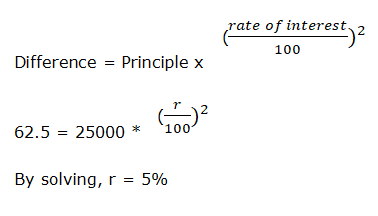# IBPS Clerk Prelims Quantitative Aptitude 2021 (Day-04)

Dear Aspirants, Our IBPS Guide team is providing new series of Quantitative Aptitude Questions for IBPS Clerk Prelims 2021 so the aspirants can practice it on a daily basis. These questions are framed by our skilled experts after understanding your needs thoroughly. Aspirants can practice these new series questions daily to familiarize with the exact exam pattern and make your preparation effective.

### Start Quiz

Approximation

Directions (01-05): What approximate value should come in the place of (?) in the following questions.

1) √255 + √1370 + √575 = ? * 7.02

A.13

B.11

C.16

D.20

E.8

2) 20.24 % of 419.93 + 14.92 * 12.13 = ?

A.268

B.250

C.264

D.256

E.278

3) 59.98 % of 119.89 + ? = 32.98 * 3.99

A.90

B.40

C.50

D.60

E.80

4) 14.28 % of 104.97 + √399 = ? – 79.95

A.115

B.135

C.145

D.125

E.105

5) 83.33 % of 479.92 + 24.93 % of 39.89 = ? * 9.99

A.31

B.37

C.41

D.48

E.55

Train

6) The length of platform is 50% more than the length of the train A and the speed of the train A is 90 kmph. The ratio of the length of train A and B is 4:3 and the speed of the train B is 60 kmph. If the train A crosses a platform in 40 seconds, then what is the time taken by train A crosses train B is running in the same direction?

A.84 seconds

B.78 seconds

C.96 seconds

D.120 seconds

E.None of these

SI AND CI

7) The difference between Simple interest and Compound interest accrued on a sum of 25000 at the same rate of interest for 2 years is 62.5. Find the rate of interest.

A.10%

B.15%

C.20%

D.5%

E.7.5%

Mensuration

8) Volume of the cuboid is 3240 cm3 and the ratio of the length, height and breadth of the cuboid is 6:5:4. If the height of the cuboid is equal to the side of the cube, then what is the surface area of the cube?

A.1650 cm2

B.1860 cm2

C.1290 cm2

D.1350 cm2

E.None of these

Ages

9) The average ages of Divya and Esai is 27 years and the average ages of Seetha, Divya and Esai is 24 years. If the average age of Arul, Banu and Seetha is 20 years, then find the average ages of Arul and Banu?

A.20 years

B.21 years

C.23 years

D.24 years

E.27 years

Mensuration

10) The ratio of the radius of cone to cylinder is 1:2 and the ratio of the height of the cone to cylinder is 4:3. What is the ratio of the volume of cone to Cylinder?

A.2:7

B.2:5

C.1:6

D.1:9

E.3:7

### Try IBPS Clerk Prelims Mock Test

√255 + √1370 + √575 = ? * 7.02

16 + 37 + 24 = ? * 7

11 = ?

20.24 % of 419.93 + 14.92 * 12.13 = ?

84 + 180 = ?

? = 264

59.98 % of 119.89 + ? = 32.98 * 3.99

72 + ? = 132

? = 60

14.28 % of 104.97 + √399 = ? – 79.95

(1/7) * 105 + 20 = ? – 80

115 = ?

83.33 % of 479.92 + 24.93 % of 39.89 = ? * 9.99

(5/6) * 480 + (1/4) * 40 = ? * 10

41 = ?

Length of train A = 4x

Length of train B = 3x

Length of platform = 4x * 150/100 = 6x

10x =90 * 5/18 * 40

x = 100

Length of train A = 4 * 100 = 400 m

Length of train B = 3 * 100 = 300 m

Required time = (400 + 300)/((90 – 60) * 5/18)

= 84 seconds6x * 5x * 4x = 3240

x = 3

Height of the cuboid = 5 * 3 = 15 cm

SA of the cube = 6 * 15 * 15 = 1350 cm2

Divya + Esai = 27 * 2 = 54

Divya + Esai + seetha = 24 * 3 = 72

Seetha = 72 – 54 = 18 years

Arul + Banu + seetha = 20 * 3 = 60

Arul + Banu = 60 – 18 = 42 years

Required average = 42/2 = 21 years

Radius of the cone = x

Radius of the cylinder = 2x

Height of cone = 4y

Height of cylinder = 3y

Required ratio = 1/3 * 22/7 * x * x * 4y: 22/7 * 2x * 2x * 3y

= 1:9

### Download IBPS Clerk Prelims Quants Free Practice Set Day 4 PDF

#### For Day Wise IBPS Clerk Practice Questions

Get Quantitative Aptitude Study Materials

Get All Section Study Materials